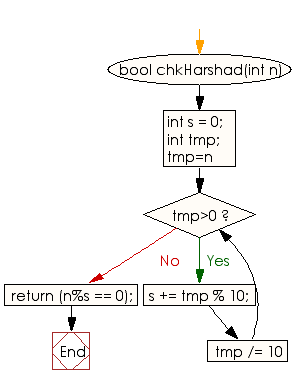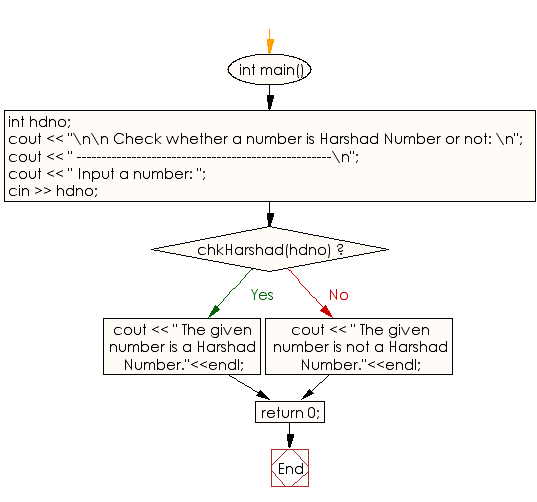﻿ C++ Exercises: Check if a number is Harshad Number or not - w3resource

# C++ Exercises: Check if a number is Harshad Number or not

## C++ Numbers: Exercise-20 with Solution

Write a C++ program to check if a number is a Harshad Number or not.

Sample Solution:

C++ Code :

``````#include<bits/stdc++.h>
using namespace std;
{
int s = 0;
int tmp;
for (tmp=n; tmp>0; tmp /= 10)
s += tmp % 10;
return (n%s == 0);
}

int main()
{
int hdno;
cout << "\n\n Check whether a number is Harshad Number or not: \n";
cout << " ---------------------------------------------------\n";
cout << " Input a number: ";
cin >> hdno;

cout << " The given number is a Harshad Number."<<endl;
else
cout << " The given number is not a Harshad Number."<<endl;
return 0;

}
``````

Sample Output:

```Check whether a number is Harshad Number or not:
---------------------------------------------------
Input a number: 18
The given number is a Harshad Number.
```

Flowchart:C++ Code Editor: